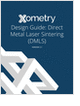MaterialsDesign CenterProcessesUnit ConversionFormulasMathematicsCalculatorsDiscussion ForumTrade PublicationsDirectory Service
Metal 3D Printing Design Guide

Direct Metal Laser Sintering (DMLS) 3D printing for parts with reduced cost and little waste.

Injection Molding Design Guide

Guide for high quality and cost-effective plastic injection molding.

3D Scanners

A white paper to assist in the evaluation of 3D scanning hardware solutions.

CNC Machining Design Guide

more free magazinesGlossary » Units » Power (Heat Flow) » Horsepower (boiler)Horsepower (boiler) (HP) is a unit in the category of Power (Heat flow). It is also known as horsepowers. This unit is commonly used in the US unit system. Horsepower (boiler) (HP) has a dimension of ML2T-3 where M is mass, L is length, and T is time. It can be converted to the corresponding standard SI unit W by multiplying its value by a factor of 9809.5.
Note that the seven base dimensions are M (Mass), L (Length), T (Time), Q (Temperature), N (Aamount of Substance), I (Electric Current), and J (Luminous Intensity).

Other units in the category of Power (Heat flow) include Abwatt (emu of Power) (aW), British Thermal Unit (IT) Per Hour (Btu (IT)/h), British Thermal Unit (IT) Per Minute (Btu (IT)/min), British Thermal Unit (IT) Per Second (Btu (IT)/s), British Thermal Unit (therm.) Per Hour (Btu (therm.)/h), British Thermal Unit (therm.) Per Minute (Btu (therm.)/min), British Thermal Unit (therm.) Per Second (Btu (therm.)/s), Calorie (IT) Per Hour (cal (IT)/h), Calorie (IT) Per Minute (cal (IT)/min), Calorie (IT) Per Second (cal (IT)/s), Calorie (therm.) Per Hour (cal (therm.)/h), Calorie (therm.) Per Minute (cal (therm.)/min), Calorie (therm.) Per Second (cal (therm.)/s), Cheval-Vapeur (horsepower) (cv, HP), Dyne-Centimeter Per Second (dyn-cm/s), Erg Per Second (erg/s), Foot-Pound Force Per Hour (ft-lbf/h), Foot-Pound Force Per Minute (ft-lbf/min), Foot-Pound Force Per Second (ft-lbf/s), Foot-Poundal Per Second (ft-pdl/s), Horsepower (550 Ft-Lbf/s) (HP), Horsepower (British) (BHP, hp), Horsepower (cheval-Vapeur) (cv, HP), Horsepower (electric) (HP), Horsepower (metric) (HP), Horsepower (water) (HP), Kilogram Force-Meter Per Second (kgf-m/s), Kilowatt (kW), Prony, Ton (refrigeration, UK) (CTR (UK)), Ton (refrigeration, US) (CTR (US)), Volt-Ampere (VA), Watt (W), Watt (int. Mean) (W), and Watt (int. US) (W).N/ARelated PagesMetal 3D Printing Design Guide

Direct Metal Laser Sintering (DMLS) 3D printing for parts with reduced cost and little waste.Injection Molding Design Guide

Guide for high quality and cost-effective plastic injection molding.3D Scanners

A white paper to assist in the evaluation of 3D scanning hardware solutions.CNC Machining Design Guide